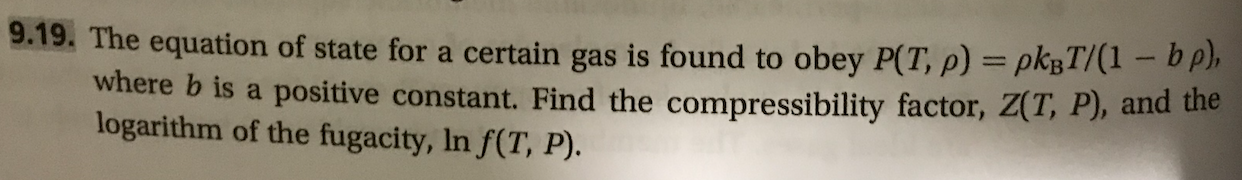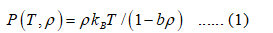# 9.19. The equation of state for a certain gas is found to obey P(T, p)-ρ㎏ld-bpFind the compressibility factor, Z(T, P), and thewhere b is a positive constant.logarithm of the fugacity, In f(T, P).

Question
84 views

This problem is (9.19) from a book  "Thermodynamics and Statistical Mechanics An Integrated Approach by M. Scott Shell"help_outlineImage Transcriptionclose9.19. The equation of state for a certain gas is found to obey P(T, p)-ρ㎏ld-bp Find the compressibility factor, Z(T, P), and the where b is a positive constant. logarithm of the fugacity, In f(T, P). fullscreen
check_circle

Step 1

The equation of the state of a certain gas is given by equation (1), where,

T represents temperature of the gas.

P represents pressure of the gas.

ρ represents molar density of the gas.

kB is the isothermal compressibility.

b is a positive constant.Step 2

The compressibility factor is a correction factor used to determine the volume of a real gas. It is the ratio of volumes of a real and ideal gas, and is given as follows:

Step 3

For 1 mol of an ideal gas, the expression for Videal is determined from the ideal gas equation as shown:

Videal = RT/P                                                                      &nb...

### Want to see the full answer?

See Solution

#### Want to see this answer and more?

Solutions are written by subject experts who are available 24/7. Questions are typically answered within 1 hour.*

See Solution
*Response times may vary by subject and question.
Tagged in

### Chemical Engineering